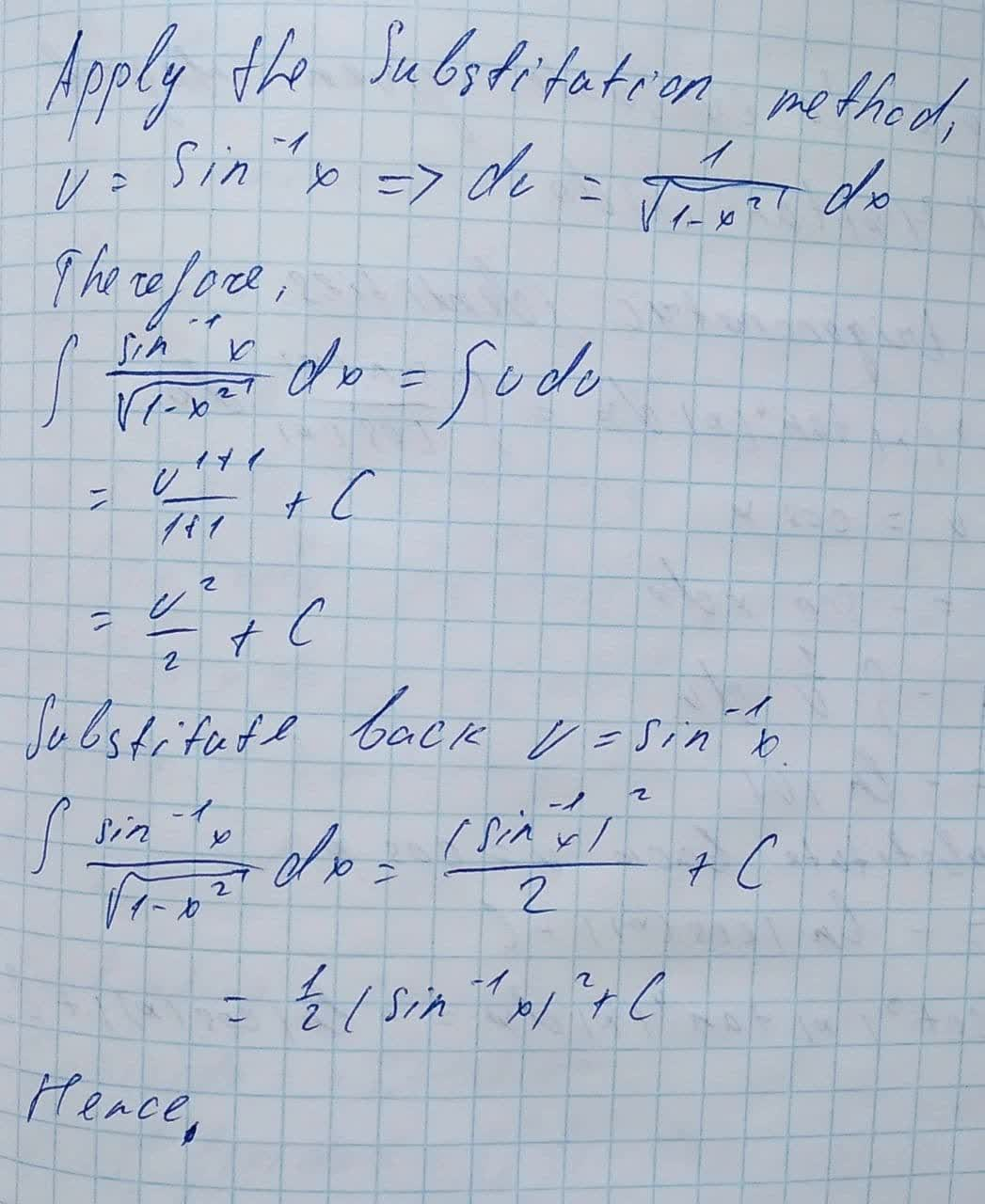Question# Evaluate the integrals: \int \frac{\sin^{-1}x}{\sqrt{1-x^{2}}}dx

Applications of integrals
ANSWEREDEvaluate the integrals.
$$\displaystyle\int{\frac{{{{\sin}^{{-{1}}}{x}}}}{{\sqrt{{{1}-{x}^{{{2}}}}}}}}{\left.{d}{x}\right.}$$